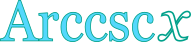## Inverse cosecant calculator

This tool evaluates the inverse cosecant of a number: arccsc(x). The principal branch is evaluated, where the return values range between -π/2 and π/2.

 x = Result: arccsc(x) = deg rad## Definitions

### General

The inverse cosecant function, in modern notation written as arccsc(x), gives the angle θ, so that:

Due to the periodical nature of the cosecant function, there are many angles θ that can give the same cosecant value (i.e. θ+2π, θ+4π, etc.). As a result, it is impossible to define a single inverse function, unless the range of the return values is restricted, so that a one-to-one relationship between θ and cscθ can be established. Therefore, multiple branches of the arccsc function can be defined. Commonly, the desired range of θ values spans between -π/2 and π/2. The branch of arccsc, in that case, is called the principal branch.

### Series

The arccsc function can be defined in a Taylor series form, like this:

The above series is valid for |x|≥1.

### Properties

The derivative of the arccsc function is:

The integral of the arccsc function is given by:

The following properties are also valid for the arccsc function: